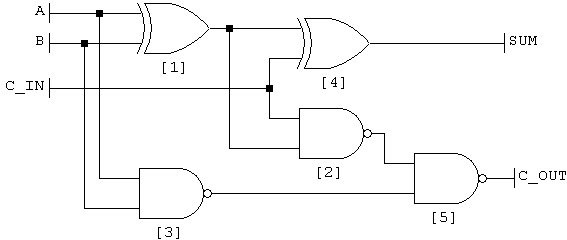## Project 6

Project Assignment (Spring 2010)

• ALU Arithmetic
• Appendix C from the CD for Computer Organization and Design, 4th Edition
• Pages C-26 to C-36:
• Simple 1-bit ALU: C-32

### VHDL Basics:

• Using VHDL, you can write code describing functional blocks made from gates. You can connect blocks together to make more complex circuits.
• Each block follows a simple pattern. Here's an example.
1. -- Two hyphens start a single-line comment
2.
3. -- These lines tell what libary to use.
4. -- You must include them before EACH entity
5. -- EVEN if you have multiple entities in the same file
6. library IEEE;
7. use IEEE.std_logic_1164.ALL;
8.
9.
10. -- This is an "entity" declaration.
11. -- Like a function declaration, it defines the inputs and outputs of the entity
13.   port (in1,in2       : in std_logic;   -- These are inputs
14.         c_in          : in std_logic;   -- This is another input
15.         sum, c_out    : out std_logic   -- These are outputs
16.         );
18.
19. -- This in an architecture declaration.  Like the body of a function, it defines how the
20. -- entity actually works. There are two types of architecture, behavioral or structural.
21. -- Behavioral architecture uses gates to define an entity.
22. -- Structural architecture connects other entities together to define an entity.  It DOES NOT use any gates on their own.
23. architecture behavioral of full_adder is
24.
25.     -- These are "signals": Internal wires that are used to interconnect gates.
26.     signal s1, s2, s3 : std_logic;
27.
28.     -- These are Time constants which I use to set delays.  You don't have to use these.
29.     constant xor_delay : Time := 3 ns;
30.     constant nand2_delay : Time := 2 ns;
31.
32. begin
33.     -- This is an xor gate with inputs in1 and in2, and output s1.
34.     s1 <= (in1 xor in2) after xor_delay;
35.
36.     -- Similarly, this is a nand gate. It has a delay of nand2_delay.
37.     -- I could have used "2 ns" instead of "nand2_delay".  They mean the same thing.
38.     s2 <= (c_in nand s1) after nand2_delay;
39.
40.     s3 <= (in1 nand in2) after nand2_delay;
41.     sum <= (s1 xor c_in) after xor_delay;
42.     c_out <= (s2 nand s3) after nand2_delay;
43. end architecture behavioral;
• When you turn in your code, you're supposed to use only one vhld file. To make this work right, you MUST put your entities in the correct order. If one of your entities uses another entity as a component, the component must come before the other in your file. This means that you'll end up with your full adder at the top of the file, and the testbench code at the very bottom. Note that you MUST put the libary declaration before each entity. This means it will appear multiple times in your file.

### Design steps:

1. Make a 1-bit "full adder". There is an example in the VHDL book. You can also find examples online.
Below is one possible gate diagram. It uses only NAND and XOR gates, which are both in your "gate libary"Don't tell anyone, but the VHDL example 'happens' to be an implementation of this circuit.
2. Now you need to make a 1-bit ALU. It needs to perform the following operations:
20010subSubtract B from A
30011subuSubtract using unsigned numbers
40100andBitwise AND
50101orBitwise OR
60110xorBitwise XOR
70111norBitwise NOR
a1010sltSet (output) 1 if A is less than B
b1011sltuSlt with unsigned numbers

This is easier than it looks. Here's the declaration for my ALU:
entity alu_1bit is
port (a,b : in std_logic;     -- operands to add/subtract/etc
cin : in std_logic;     -- carry in, needed to chain multiple ALUs together
less : in std_logic;    -- this is the input that determines the result of slt and sltu.
--It's technically only needed in the lowest-order bit (bit 0) of the ALU
op  : in std_logic_vector(3 downto 0);  -- opcode input, 4 bits
z   : out std_logic             -- ALU output (the 'answer')
cout    : out std_logic         -- carry out
-- Technically only needed for the highest-order bit (bit 3)
ovf : out std_logic             -- Overflow output.  Only needed for the highest-order bit
);
end alu_1bit;
• The instructions tell you that you'll need special ALUs for the highest and lowest order bits. I've added the 'special' features to my normal 1-bit ALU so that I only need one kind.
• Each of the bitwise operations is trivial. XOR and NOR are in your gate libary, so start by making signals containing A XOR B and A NOR B. NAND is the closest we have to AND, so also make a signal with A NAND B. Remember to use appropriate gate delays.
• For the other operations we'll need to use our full adder. A few notes about these operations:
• There is no difference in the ALU's answer for "signed" or "unsigned" operations. The only difference is in the overflow detection.
• To subtract B from A, we use a trick of two's complement arithmetic: A - B is the same as A + NOT(B) + 1.
• First we'll need to use B for add and the bitwise operations, and NOT(B) for subtract and slt(u). First make a signal called notB containing NOT(B). Then use the when statement to make a multiplexer:
invb <= b when "0000", -- add